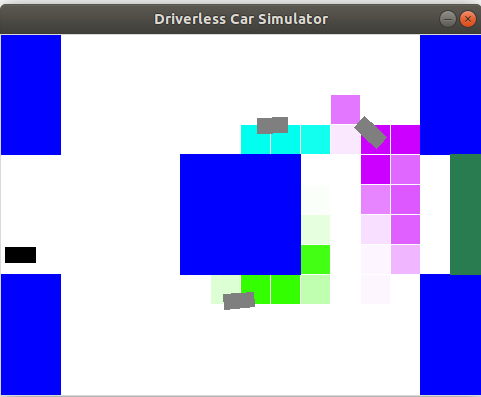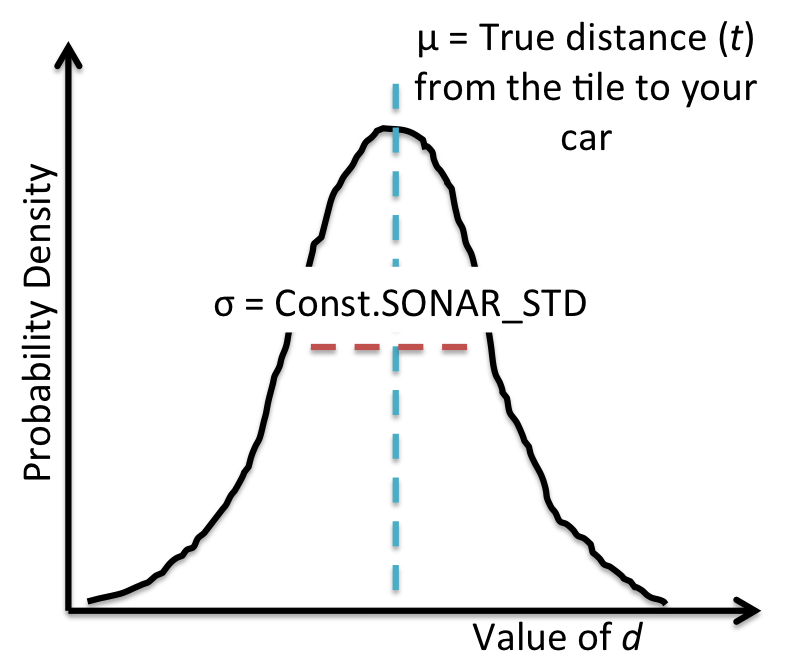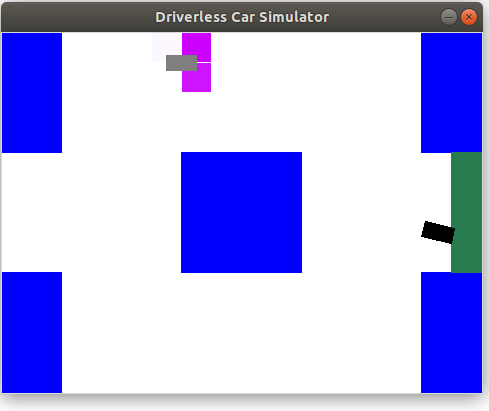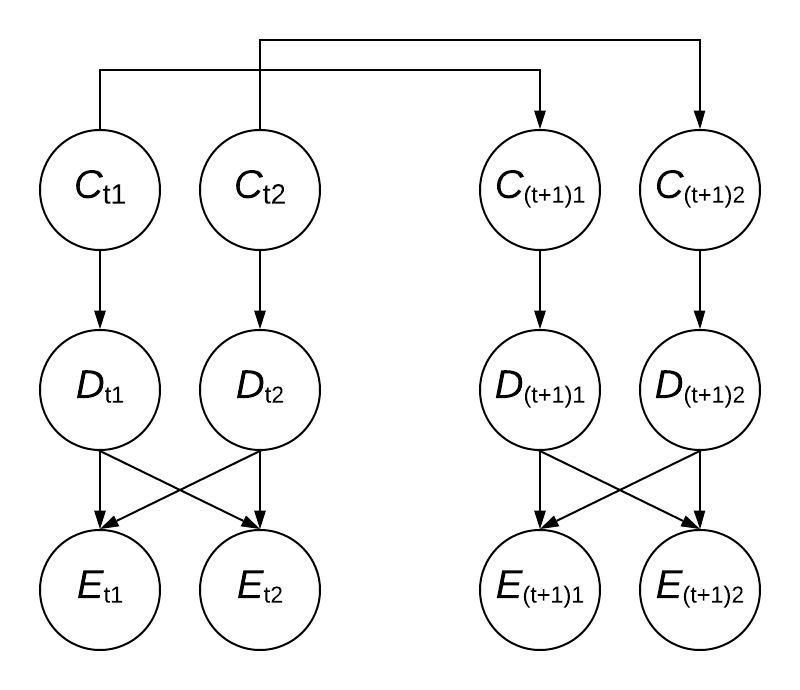We've created a LaTeX template here for you to use that contains the prompts for each question.This assignment is a modified version of the Driverless Car assignment written by Chris Piech.

A study by the World Health Organization found that road accidents kill a shocking 1.24 million people a year worldwide. In response, there has been great interest in developing autonomous driving technology that can drive with calculated precision and reduce this death toll. Building an autonomous driving system is an incredibly complex endeavor. In this assignment, you will focus on the sensing system, which allows us to track other cars based on noisy sensor readings.

Getting started. You will be running two files in this assignment - grader.py and drive.py. The drive.py file is not used for any grading purposes, it's just there to visualize the code you will be writing and help you gain an appreciation for how different approaches result in different behaviors (and to have fun!). Let's start by trying to drive manually.

python drive.py -l lombard -i none

You can steer by either using the arrow keys or 'w', 'a', and 'd'. The up key and 'w' accelerates your car forward, the left key and 'a' turns the steering wheel to the left, and the right key and 'd' turns the steering wheel to the right. Note that you cannot reverse the car or turn in place. Quit by pressing 'q'. Your goal is to drive from the start to finish (the green box) without getting in an accident. How well can you do on the windy Lombard street without knowing the location of other cars? Don't worry if you're not very good; the teaching staff were only able to get to the finish line 4/10 times. An accident rate of 60% is pretty abysmal, which is why we're going to use AI to do this.

Flags for python drive.py:

• -a: Enable autonomous driving (as opposed to manual).
• -i <inference method>: Use none, exactInference, particleFilter to (approximately) compute the belief distributions over the locations of the other cars.
• -l <map>: Use this map (e.g. small or lombard). Defaults to small.
• -d: Debug by showing all the cars on the map.
• -p: All other cars remain parked (so that they don't move).

Modeling car locations

We assume that the world is a two-dimensional rectangular grid on which your car and $K$ other cars reside. At each time step $t$, your car gets a noisy estimate of the distance to each of the cars. As a simplifying assumption, we assume that each of the $K$ other cars moves independently and that the noise in sensor readings for each car is also independent. Therefore, in the following, we will reason about each car independently (notationally, we will assume there is just one other car).

At each time step $t$, let $C_t \in \mathbb R^2$ be a pair of coordinates representing the actual location of the single other car (which is unobserved). We assume there is a local conditional distribution $p(c_t \mid c_{t-1})$ which governs the car's movement. Let $a_t \in \mathbb R^2$ be your car's position, which you observe and also control. To minimize costs, we use a simple sensing system based on a microphone. The microphone provides us with $D_t$, which is a Gaussian random variable with mean equal to the true distance between your car and the other car and variance $\sigma^2$ (in the code, $\sigma$ is Const.SONAR_STD, which is about two-thirds the length of a car). In symbols,

$D_t \sim \mathcal N(\|a_t - C_t\|_2, \sigma^2)$.

For example, if your car is at $a_t = (1,3)$ and the other car is at $C_t = (4,7)$, then the actual distance is $5$ and $D_t$ might be $4.6$ or $5.2$, etc. Use util.pdf(mean, std, value) to compute the probability density function (PDF) of a Gaussian with given mean mean and standard deviation std, evaluated at value. Note that evaluating a PDF at a certain value does not return a probability -- densities can exceed $1$ -- but for the purposes of this assignment, you can get away with treating it like a probability. The Gaussian probability density function for the noisy distance observation $D_t$, which is centered around your distance to the car $\mu = \|a_t - C_t\|_2$, is shown in the following figure:Your job is to implement a car tracker that (approximately) computes the posterior distribution $\mathbb P(C_t \mid D_1 = d_1, \dots, D_t = d_t)$ (your beliefs of where the other car is) and update it for each $t = 1, 2, \dots$. We will take care of using this information to actually drive the car (i.e., set $a_t$ to avoid a collision with $c_t$), so you don't have to worry about that part.

To simplify things, we will discretize the world into tiles represented by (row, col) pairs, where 0 <= row < numRows and 0 <= col < numCols. For each tile, we store a probability representing our belief that there's a car on that tile. The values can be accessed by: self.belief.getProb(row, col). To convert from a tile to a location, use util.rowToY(row) and util.colToX(col).

Here's an overview of the assignment components:

• In Problems 1 and 2 (code), you will implement ExactInference, which computes a full probability distribution of another car's location over tiles (row, col).
• In Problem 3 (code), you will implement ParticleFilter, which works with particle-based representation of this same distribution.
• Problem 4 (written) gives you a chance to extend your probability analyses to a slightly more realistic scenario where there are multiple other cars and we can't automatically distinguish between them.

A few important notes before we get started:

• Past experience suggests that this will be one of the most conceptually challenging assignments of the quarter for many students. Please start early, especially if you're low on late days!
• We strongly recommend that you attend/watch the lectures on Bayesian networks and HMMs before getting started, and keep the slides handy for reference while you're working.
• The code portions of this assignment are short and straightforward -- no more than about 30 lines in total -- but only if your understanding of the probability concepts is clear! (If not, see the previous point.)
• As a notational reminder: we use the lowercase expressions $p(x)$ or $p(x|y)$ for local conditional probability distributions, which are defined by the Bayesian network. We use the uppercase expressions $\mathbb P(X = x)$ or $\mathbb P(X = x | Y = y)$ for joint and posterior probability distributions, which are not pre-defined in the Bayesian network but can be computed by probabilistic inference. Please review the lecture slides for more details.

Problem 1: Emission probabilities

In this problem, we assume that the other car is stationary (e.g., $C_t = C_{t-1}$ for all time steps $t$). You will implement a function observe that upon observing a new distance measurement $D_t = d_t$ updates the current posterior probability from $$\mathbb P(C_t \mid D_1 = d_1, \dots, D_{t-1} = d_{t-1})$$ to $$\mathbb P(C_t \mid D_1 = d_1, \dots, D_t = d_t) \propto \mathbb P(C_t \mid D_1 = d_1, \dots, D_{t-1} = d_{t-1}) p(d_t \mid c_t),$$ where we have multiplied in the emission probabilities $p(d_t \mid c_t)$ described earlier under "Modeling car locations". The current posterior probability is stored as self.belief in ExactInference.1. Fill in the observe method in the ExactInference class of submission.py. This method should modify self.belief in place to update the posterior probability of each tile given the observed noisy distance to the other car. After you're done, you should be able to find the stationary car by driving around it (using the flag -p means cars don't move):

Notes:

• Once you implement the observe function, you can start driving with exact inference.
python drive.py -a -p -d -k 1 -i exactInference
You can also turn off -a to drive manually.
• Read through the code in util.py for the Belief class before you get started... you'll need to use this class for several of the code tasks in this assignment.
• Remember to normalize the posterior probability after you update it. (There's a useful function for this in util.py).
• On the small map, the autonomous driver will sometimes drive in circles around the middle block before heading for the target area. In general, don't worry too much about the precise path the car takes. Instead, focus on whether your car tracker correctly infers the location of other cars.
• Don't worry if your car crashes once in a while! Accidents do happen, whether you are human or AI. However, even if there was an accident, your driver should have been aware that there was a high probability that another car was in the area.

Problem 2: Transition probabilities

Now, let's consider the case where the other car is moving according to transition probabilities $p(c_{t+1} \mid c_t)$. We have provided the transition probabilities for you in self.transProb. Specifically, self.transProb[(oldTile, newTile)] is the probability of the other car being in newTile at time step $t+1$ given that it was in oldTile at time step $t$.

In this part, you will implement a function elapseTime that updates the conditional probability about the location of the car at a current time $t$ $$p(C_t = c_t \mid D_1 = d_1, \dots, D_t = d_t)$$ to the next time step $t+1$ conditioned on the same evidence, via the recurrence: $$p(C_{t+1} = c_{t+1} \mid D_1 = d_1, \dots, D_t = d_t) \propto \sum_{c_t} p(C_t = c_t \mid D_1 = d_1, \dots, D_t = d_t) p(c_{t+1} \mid c_t).$$ Again, the posterior probability is stored as self.belief in ExactInference.

1. Finish ExactInference by implementing the elapseTime method. When you are all done, you should be able to track a moving car well enough to drive autonomously by running the following.
2. python drive.py -a -d -k 1 -i exactInference

Notes:

• You can also drive autonomously in the presence of more than one car:
python drive.py -a -d -k 3 -i exactInference
• You can also drive down Lombard:
python drive.py -a -d -k 3 -i exactInference -l lombard
On Lombard, the autonomous driver may attempt to drive up and down the street before heading towards the target area. Again, focus on the car tracking component, instead of the actual driving.

Problem 3: Particle filtering

Though exact inference works well for the small maps, it wastes a lot of effort computing probabilities for every available tile, even for tiles that are unlikely to have a car on them. We can solve this problem using a particle filter. Updates to the particle filter have complexity that's linear in the number of particles, rather than linear in the number of tiles.

For a great conceptual explanation of how particle filtering works, check out this video on using particle filtering to estimate an airplane's altitude.

In this problem, you'll implement two short but important methods for the ParticleFilter class in submission.py. When you're finished, your code should be able to track cars nearly as effectively as it does with exact inference.

1. Some of the code has been provided for you. For example, the particles have already been initialized randomly. You need to fill in the observe and elapseTime functions. These should modify self.particles, which is a map from tiles (row, col) to the number of particles existing at that tile, and self.belief, which needs to be updated each time you re-sample the particle locations.

You should use the same transition probabilities as in exact inference. The belief distribution generated by a particle filter is expected to look noisier compared to the one obtained by exact inference.

python drive.py -a -i particleFilter -l lombard
To debug, you might want to start with the parked car flag (-p) and the display car flag (-d).

Note: The random number generator inside util.weightedRandomChoice behaves differently on different systems' versions of Python (e.g., Unix and Windows versions of Python). We ask that you run the code locally using Python 3.7 for consistency with the autograder.

Problem 4: Which car is it?

So far, we have assumed that we have a distinct noisy distance reading for each car, but in reality, our microphone would just pick up an undistinguished set of these signals, and we wouldn't know which distance reading corresponds to which car. First, let's extend the notation from before: let $C_{ti} \in \mathbb R^2$ be the location of the $i$-th car at the time step $t$, for $i = 1, \dots, K$ and $t = 1, \dots, T$. Recall that all the cars move independently according to the transition dynamics as before.

Let $D_{ti} \in \mathbb R$ be the noisy distance measurement of the $i$-th car at time step $t$, which is now not directly observed. Instead, we observe the unordered set of distances $\{ D_{t1}, \dots, D_{tK} \}$ as a collective and so cannot attribute any individual measurement in this set to a specific car. (For simplicity, we'll assume that all distances are distinct values.) In other words, you can think of this scenario as the same as observing the list $\mathbf{E_t} = [E_{t1}, \dots, E_{tK}]$ which is a uniformly random permutation of the (noisy) correctly ordered distances $\mathbf{D_t} = [D_{t1}, \dots, D_{tK}]$ where index $i$ represents the noisy distance to car $i$ at time $t$.

For example, suppose $K=2$ and $T = 2$. Before, we might have gotten distance readings of $8$ and $4$ for the first car and $5$ and $7$ for the second car at time steps $1$ and $2$, respectively. Now, our sensor readings would be permutations of $\{8, 5\}$ (at time step $1$) and $\{4, 7\}$ (at time step $2$). Thus, even if we knew the second car was distance $5$ away at time $t = 1$, we wouldn't know if it moved further away (to distance $7$) or closer (to distance $4$) at time $t = 2$.Here is a diagram that shows the flow of information corresponding to the above situation for the case where $K = 2$ and only showing two timesteps, $t$ and $t+1$. Note that because the observed distances $\mathbf{E_t}$ are a permutation of the true distances $\mathbf{D_t}$, each $E_{ti}$ depends on all of the $D_{ti}$. Also note that the above diagram is not a Bayes net as $E_{t1}$ and $E_{t2}$ are not conditionally independent given $D_{t1}$ and $D_{t2}$ (however, $D_{t1}$ and $D_{t2}$ are conditionally independent given $C_{t1}$ and $C_{t2}$)

1. Suppose we have $K=2$ cars and one time step $T=1$. Write an expression for the conditional probability $p(C_{11} = c_{11}, C_{12} = c_{12} \mid \mathbf{E_1} = \mathbf{e_1})$ as a function of the PDF of a Gaussian $\mathcal p_{\mathcal N}(v; \mu, \sigma^2)$ and the prior probability $p(c_{11})$ and $p(c_{12})$ over car locations. Your final answer should not contain variables $D_{11}$, $D_{12}$.

Remember that $\mathcal p_{\mathcal N}(v; \mu, \sigma^2)$ is the probability of a random variable, $v$, in a Gaussian distribution with mean $\mu$ and standard deviation $\sigma$.

Hint: for $K=1$, the answer would be $$p(C_{11} = c_{11} \mid \mathbf{E_1} = \mathbf{e_1}) \propto p(c_{11}) p_{\mathcal N}(e_{11}; \|a_1 - c_{11}\|_2, \sigma^2).$$ where $a_t$ is the position of your car (that you are controlling) at time $t$. Remember that $C_{ti}$ is the position of the $i$th observed car at time $t$. To better inform your use of Bayes' rule, you may find it useful to draw the Bayesian network and think about the distribution of $\mathbf{E_t}$ given $D_{t1}, \dots, D_{tK}$.

Hint: Note that the observed variable(s) are the shuffled/randomized distances $\mathbf{E_t} = [E_{t1}, E_{t2}, ..., E_{tK}]$. These are a random permutation of the unobserved noisy distances $\mathbf{D_t} = [D_{t1}, D_{t2}, ..., D_{tK}]$, where $D_{t1}$ is the distance of car $1$ at timestep $t$. Note that $E_{t1}$ is the emission from one of the cars at timestep $t$, but we aren't sure which one (it is NOT necessarily car 1, it could be any of the cars). On the other hand, $D_{t1}$ is the measured distance of car 1 (we know with certainty that it comes from car 1), the only issue is that we don't observe it directly.

Hint: To reduce notation, you may write, for example, $p(c_{11}∣e_{11})$ instead of $p(C_{11}=c_{11}∣E_{11}=e_{11})$.

A mathematical expression, with the steps you took to derive that expression, relating (can be proportionality) $p(C_{11} = c_{11}, C_{12} = c_{12} | \mathbf{E_1} = \mathbf{e_1})$ with the PDF of a Gaussian and the priors $p(c_{11})$ and $p(c_{12})$ over car locations.
2. Assuming the prior $p(c_{1i})$ of where the cars start out is the same for all $i$ (i.e. for all K cars), show that the number of assignments for all $K$ cars $(c_{11}, \dots, c_{1K})$ that obtain the maximum value of $p(C_{11} = c_{11}, \dots, C_{1K} = c_{1K} \mid \mathbf{E_1} = \mathbf{e_1})$ is at least $K!$ (K factorial).

You can also assume that the car locations that maximize the probability above are unique ($c_{1i} \neq c_{1j}$ for all $i \neq j$).

Hint: The priors $p(c_{1i})$ are a probability distribution over the possible starting positions ($t=1$) of each car $i$. Note that even if the car positions share the same prior, it doesn't necessarily mean they have the exact same start positions $c_{1i}$ because the start positions are sampled from the prior distribution, which can yield different values each time it is sampled from. However, you should think about what the priors all being the same means intuitively in terms of how we can associate observations with cars.

Note: you don't need to produce a complicated proof for this question. It is acceptable to provide a clear explanation based on your intuitive understanding of the scenario.

Either a short mathematical argument or concise explanation for why the statement defined in the problem is true.
3. (extra credit) For general $K$, what is the treewidth corresponding to the posterior probability over all $K$ car locations at all $T$ time steps conditioned on all the sensor readings: $$p(C_{11} = c_{11}, \dots, C_{1K} = c_{1K}, \dots, C_{T1} = c_{T1}, \dots, C_{TK} = c_{TK} \mid \mathbf{E_1} = \mathbf{e_1}, \dots, \mathbf{E_T} = \mathbf{e_T})?$$ Briefly justify your answer.

For reference, the treewidth of a factor graph is defined as the maximum arity (number of variables that a factor depends on) of any factor created by variable elimination under the best variable elimination ordering. You can find further information, along with an example, that may be relevant to this problem here.

The treewidth as a function of $K$ and a brief justification for why the treewidth is represented by that function.
4. (extra credit) Now suppose you change your sensors so that at each time step $t$, they return the list of exact positions of the $K$ cars, but the list of positions is shifted by a random number of indices (with wrap around). For example, if the true car positions at time step $1$ are $c_{11} = (1, 1) , c_{12} = (3, 1), c_{13} = (8, 1), c_{14} = (5, 2)$, then $\mathbf{e_1}$ would be $[(1, 1), (3, 1), (8, 1), (5, 2)]$, $[(3, 1), (8, 1), (5, 2), (1, 1)]$, $[(8, 1), (5, 2), (1, 1), (3, 1)]$, or $[(5, 2), (1, 1), (3, 1), (8, 1)]$, each with probability $1/4$. Describe an efficient algorithm for computing $p(c_{ti} \mid \mathbf{e_1}, \dots, \mathbf{e_T})$ for any time step $t$ and car $i$. Your algorithm should not be exponential in $K$ or $T$.
A description of the factor graph/Bayesian net used to model the problem, including any relevant variables and conditional probabilities. Also, a description of how you would use the factor graph to compute the provided probability. Note that you should try to simplify your expression for the probability as much as possible given the information provided.

Problem 5: Ethics in Advanced Technologies

1. You are in charge of public policy for an autonomous vehicle company headquartered in California. Your engineering team is making progress in designing a fully automated vehicle and would like to test it on real roadways. California would require that you apply for autonomous vehicle testing permits and meet regulatory standards designed to protect the safety of other motorists and pedestrians. Given these regulations, testing your vehicles in California secretly without a permit would be illegal. The Governor of Arizona has reached out to offer your company testing in the state without restrictions. Doing so would allow you to test more quickly and without making any changes to your vehicles. On the other hand, testing in Arizona could be considered “ethics dumping,” namely “doing research deemed unethical in a scientist’s home country in a country or region with laxer ethical rules” and regulations. 

Would testing in Arizona be “ethics dumping”? Be sure to explain why you think it is or isn’t. Given your answer to this question and other factors you consider to be relevant, should you perform your tests in Arizona or comply with Californian standards? Justify your answer with a reason as to why.

In 3-6 sentences, we expect:
• a yes or no answer to the ethics dumping question
• an explanation of why it is or isn’t ethics dumping given the definition above
• a yes or no answer to whether the tests should be done in Arizona or California
• an explanation that justifies why the tests should be done in Arizona or California
2. Dual-use technologies are technologies that serve two purposes, typically a military and a civilian purpose. Researchers developing dual-use technologies face a moral dilemma: though they may intend to improve only the peaceful use of the technology, any improvements they make aid others who use the technology in war or in non-state attacks or killings. Tracking of the kind developed in this assignment can be used in self-driving cars or in autonomous weapons systems, such as lethal drones that track people to kill them.

Imagine a researcher who develops a dual-use technology despite knowing about the lethal secondary use of the technology and despite the researcher considering this secondary use unethical. Would the researcher be partially morally responsible for improvements to the lethal use of the technology that result from their discoveries? If not, give one reason why not. If so, explain why they are partially responsible and explain what action(s) the researcher should take to address this (choosing another line of research, building a safeguard, or other)?

What we expect: A 3-6 sentence response that
• answers yes or no to whether the researcher would be partially morally responsible
• provides a reason why they would or would not be partially morally responsible
• if yes, describes an action they should take

 Thanks to Rob Reich for this example. For a discussion of ethics dumping, see this

 https://www.theguardian.com/technology/2018/mar/28/uber-arizona-secret-self-driving-program-governor-doug-ducey